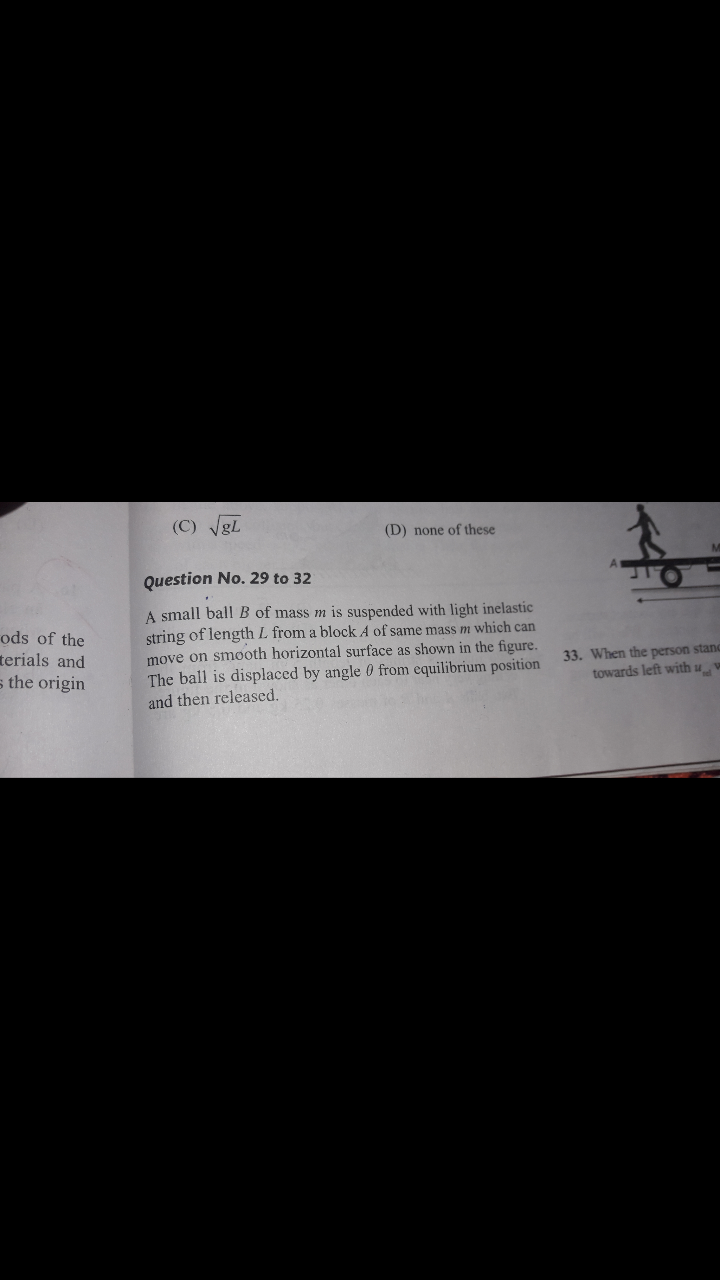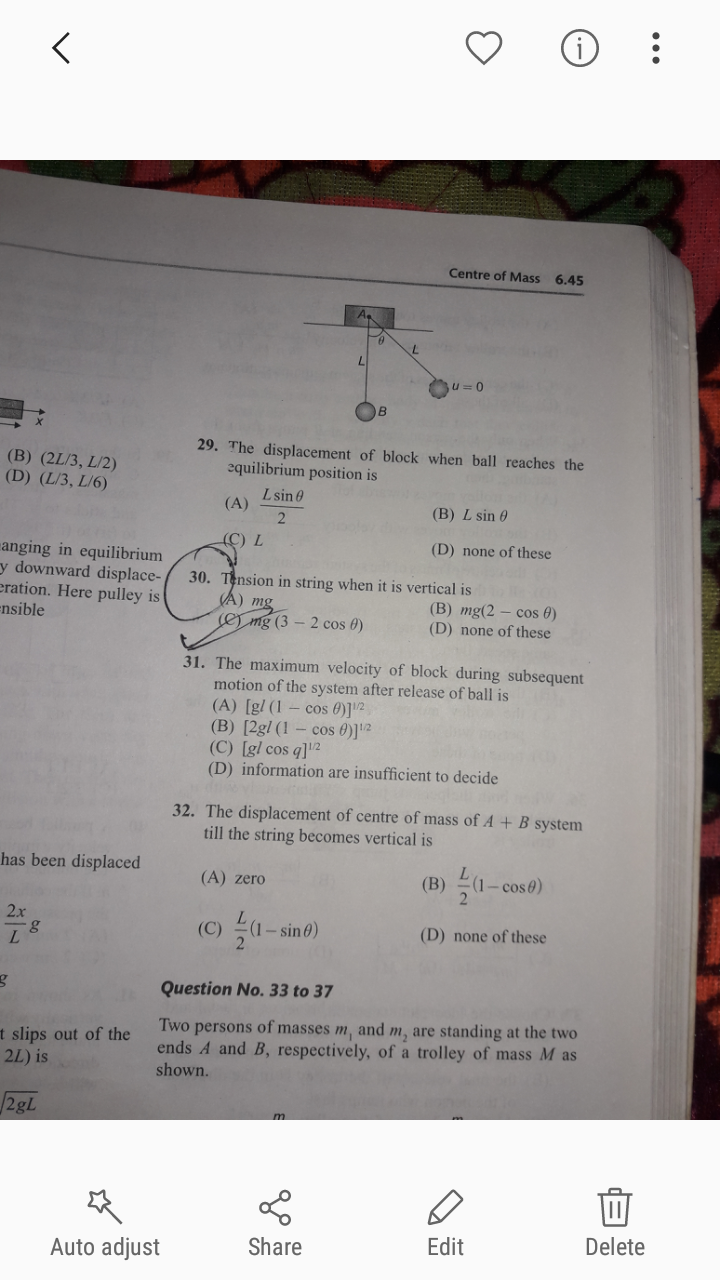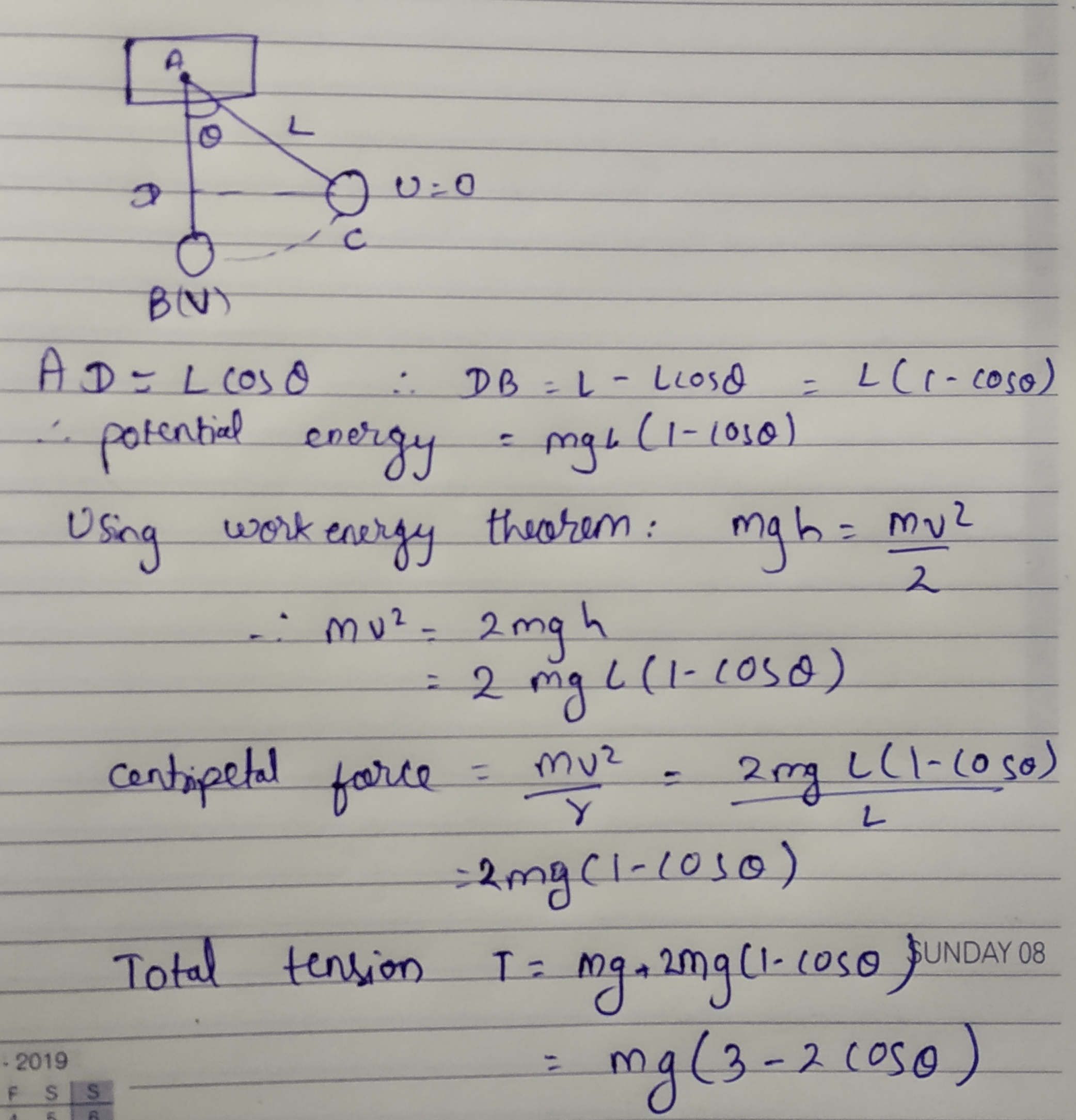# Doubt from centre of mass1st equation is derived from work energy theorem, second is the F= ma equation.

Sorry for some reason the photo is not getting uploaded.

The vertical displacement here is L(1-cos@).
Using WET, v at lowest point is √2gL(1-cos@)
Therefore centripetal acceleration at lowest point is v^2/L, which also happens to be the net acceleration a of the ball.

So, T - mg = ma, solving it you shall get the value of T

please tell what should be ans acc to you because i think my book have typo error